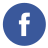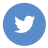### bubble sort in java

java

Bubble sort is a simple sorting algorithm that repeatedly compares adjacent elements in an array and swaps them if they are in the wrong order. It continues this process until the array is sorted in ascending or descending order.

Here is an example of how to implement bubble sort in Java:

``````public class Main {
public static void main(String[] args) {
// Create an array of integers
int[] numbers = { 5, 2, 7, 1, 4 };

// Sort the array using bubble sort
bubbleSort(numbers);

// Print the sorted array
for (int number : numbers) {
System.out.print(number + " ");
}
// prints "1 2 4 5 7 "
}

// Bubble sort method
public static void bubbleSort(int[] numbers) {
// Flag to indicate if a swap was made in the current pass
boolean swapped;

do {
// Set the flag to false
swapped = false;

// Loop through the array
for (int i = 0; i < numbers.length - 1; i++) {
// If the current element is greater than the next element, swap them
if (numbers[i] > numbers[i + 1]) {
int temp = numbers[i];
numbers[i] = numbers[i + 1];
numbers[i + 1] = temp;

// Set the flag to true
swapped = true;
}
}
} while (swapped); // Repeat the process until no swaps are made
}
}``````

This code defines a `bubbleSort()` method that uses a `do`-`while` loop to repeatedly compare and swap adjacent elements in the array until it is sorted. It then prints the sorted array to the console.

Keep in mind that bubble sort is not a very efficient sorting algorithm, especially for large arrays, and there are faster sorting algorithms available such as quicksort and mergesort.